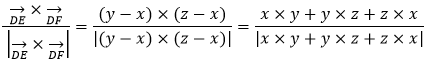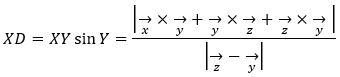# How to Find the Area of a Triangle using Vectors

In vector theory, vectors are visualized as directed line segments whose lengths are their magnitudes. We will use this concept well in this concept explanation, the area of a triangle formed by vectors. Normally when we try to find out the area of a triangle, we usually find out the value by the formula of Heron’s Formula. We can express the area of a triangle by vectors also.

We know that in a parallelogram when the two adjacent sides are given by $\vec {AB}$ and $\vec {AC}$ and the angle between the two sides are given by θ then the area of the parallelogram will be given by $|\vec {AB} \times \vec {AC}|$ and the value will be given by $|\vec {AB} | \times |\vec {AC}|$× sin⁡ θ.

A triangle divides a parallelogram into two equal parts, so the area of the triangle will be given by 1/2 x $|\vec {AB} | \times |\vec {AC}|$× sin⁡θ

## Formula

When two vectors are given:

Below are the expressions used to find the area of a triangle when two vectors are known.

1/2 x $|\vec {AB} \times \vec {AC}|$

or 1/2 x $|\vec {BC} \times \vec {CA}|$

or 1/2 x $|\vec {CB} \times \vec {CA}|$

When three vectors are given:

Expression to find the area of a triangle when three vectors will be given.

Let the sides of ∆ABC be represented by $\vec a, \vec b\ and\ \vec c$. Basically they will give us the position vectors of the corresponding sides. If they are the position vectors of the ∆ABC then the area of the triangle will be written as

1/2 × $|\vec a \times \vec b + \vec b \times \vec c + \vec c \times \vec a |$.

Some important properties:

(i) If the three vertices of a ∆ABC be presented by $\vec a, \vec b\ and\ \vec c$ then the centroid of triangle is $\frac{\vec a + \vec b + \vec c}{3}$

(ii) If the three vertices of a ∆ABC be presented by (x1, y1, z1 ),(x2, y2, z2) and (x3, y3, z3) then in that case centroid co-ordinate will be given by: $\frac{(x_{1}+ x_{2}+ x_{3})}{3},\frac{(y_{1}+ y_{2}+ y_{3})}{3},\frac{(z_{1}+ z_{2}+ z_{3})}{3}$ and

the area will be given by

Area_(yz) = 1/2$\begin{vmatrix} y_1& z_1& 1\\ y_2&z_2 &1 \\y_3 &z_3 &1 \end{vmatrix}$

Or Area_(zx) = 1/2$\begin{vmatrix} z_1& x_1& 1\\ z_2&x_2 &1 \\z_3 &x_3 &1 \end{vmatrix}$

Or Area_(xy) = 1/2$\begin{vmatrix} x_1& y_1& 1\\ x_2&y_2 &1 \\x_3 &y_3 &1 \end{vmatrix}$

(iii) If given vectors are collinear, the angle between them will be 0° and the value of sin ⁡ θ = sin⁡(0°) = 0. So, the value of the area will be zero.

## Solved Examples

Example 1: Consider a ∆ABC. O is any point inside it. The values of $\vec {OA}, \vec {OB}\ and\ \vec {OC}$ are given by

$\vec {OA}= \hat i + 3 \hat j+ 5 \hat k$ $\vec {OB}= 2 \hat i + 5 \hat j+ 7 \hat k$ and

$\vec {OC}= -3 \hat i + 10 \hat j – \hat k$

Find the area of the triangle.

Solution:

We know, area of ∆ABC = 1/2 x $|\vec {AB} \times \vec {AC}|$

So, $\vec {AB}$ = $\vec {OB} – \vec {OA}$ that is given by the difference of the position vectors of A and B.

Now, $\vec {OB} – \vec {OA} = (2 \hat i + 5 \hat j+ 7 \hat k) – (\hat i + 3 \hat j+ 5 \hat k) = \hat i + 2 \hat j+ 2 \hat k$ $\vec {AC}$ = $\vec {OC} – \vec {OA}$ that is given by the difference of the position vectors of A and C.

$\vec {OC} – \vec {OA} = (-3 \hat i + 10 \hat j – \hat k) – (\hat i + 3 \hat j+ 5 \hat k) = -4 \hat i + 7 \hat j- 6 \hat k$

Now, determine $\vec {AB} \times \vec {AC}$ which will be given by:

$\begin{vmatrix} \hat i & \hat j& \hat k \\ 1&2 &2 \\-4 &7 &-6 \end{vmatrix}$

= $\hat i(12-14) – \hat j (6-(-8) + \hat k (7-(-8) = -2\hat i – 14 \hat j + 15 \hat k$

Now, $|\vec {AB} \times \vec {AC}| = \sqrt{4 + 169 + 225}=5 \sqrt{17}$

Therefore, area of triangle = 5(√17)/2 sq. units

Example 2: If x, y and z are the position vectors for three vertices of the ∆DEF. How to represent the area of the triangle in vector form?

Solution:

The cross products of the position vectors are given by |xy + yz + zx| and the area will be given by: 1/2 |xy + yz + zx|

So, the answer will be 1/2 |xy + yz + zx|

Example 3:

If x, y and z to be the position vectors for three vertices of the ∆DEF, then show the vector form of the unit vector perpendicular to the plane of the triangle.

Solution:

If the vectors of two sides of the triangle ∆DEF be given by $\vec {DE}$ and $\vec {DF}$ then both vectors lie on the plane of the ∆DEF. So the vector $|\vec {DE} \times \vec {DF}|$ will be the unit vector which will lie perpendicular to the plane of the ∆DEF.

So, the unit vector can be represented as:Example 4: If $\vec x, \vec y\ and\ \vec z$are the position vectors of the vertices X,Y and Z of ∆XYZ, find the perpendicular distance of vertex X from the base YZ of ∆XYZ.

Solution:

We know, $|\vec {yz} \times \vec {xy}| = |\vec x \times \vec y + \vec y \times \vec z + \vec z \times \vec y|$

=> $|\vec {yz}|| \vec {xy}| sin\ Y = |\vec x \times \vec y + \vec y \times \vec z + \vec z \times \vec y|$

=> $|\vec z – \vec y|(XY sin\ Y) = |\vec x \times \vec y + \vec y \times \vec z + \vec z \times \vec y|$

So, the length of the perpendicular from X on YZ will be given by:Example 5: Find the area of the triangle with coordinates of points A (1, -1, 3), B (-2, -5, 4) and C (3, 1, -4).

Solution:

Calculate vector by initial and terminal points.

$\overline {AB} = {B_{x} – A_{x}; B_{y} – A_{y}; B_{z} – A_{z}} = {-2 – 1; -5 – (-1); 4 – 3} = {-3; -4; 1}\\ \overline {AC} = {C_{x} – A_{x}; C_{y} – A_{y}; C_{z} – A_{z}} = {3 – 1; 1 – (-1); -4 – 3} = {2; 2; -7}\\ A=\frac{1}{2}|\overline{AB}*\overline{AC}|\\$

Calculate the cross product of the vectors

$\overline {c}=\overline{AB}*\overline{AC}\\ \overline{AB}*\overline{AC}=\begin{vmatrix} i &j &k \\ AB_{x} &AB_{y} &AB_{z} \\ AC_{x}&AC_{y} &AC_{z} \end{vmatrix}\\ = i ((-4)\cdot (-7) – 1\cdot 2) – j ((-3)\cdot (-7) – 1\cdot 2) + k ((-3)\cdot 2 – (-4)\cdot 2)\\ = i (28 – 2) – j (21 – 2) + k (-6 + 8) = {26; -19; 2}\\$

Calculate the magnitude of the vector

$\overline {c}=\sqrt{{c_{x}^{2}}+{c_{y}^{2}}+{c_{z}^{2}}}\\=\sqrt{{26^{2}+[-19^{2}]+2^{2}}}\\=\sqrt{{676 + 361 + 4}}\\=\sqrt{1041}$

Calculate triangle area:

$A=\frac{1}{2}\sqrt{1041}\approx 16.132265804901678$